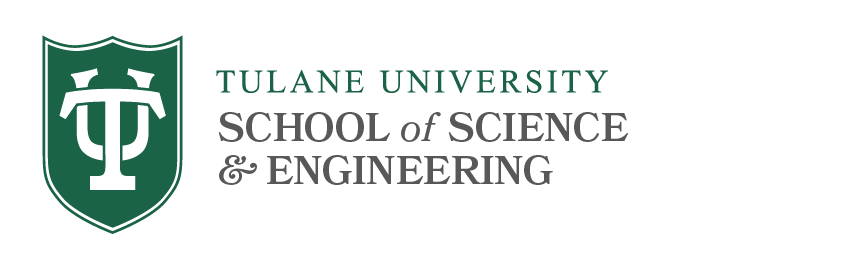# Differential Geometry Qualifying Exams

## Syllabus Topics

This exam covers the following topics:

1. Differentiable manifolds
2. Vector fields, tensors and differential forms.
3. Riemannian manifolds, connections and curvature.

On the exam you will be expected to:

• state definitions and major theorems
• be able to prove simple propositions utilizing key definitions
• do basic computations
• be able to apply main theorems to prove other results (e.g. typical homework problem with one  age proof)
• give complete proofs for more elementary results on the spot
• sketch the main outline for proofs of major results

Below we will distinguish theorems by SSA for "state, sketch the proof, and apply", SA for "state and apply" and S for "state only". The list of theorems below is not intended to be complete but the most important results are mentioned.

For each of the 3 areas, there is a primary reference which is a sufficient source of study. If you know the material from this primary reference, this is sufficient. Secondary references are also included as an additional resource. Studying from the Exercises in the provided references should be enough to pass the exam.

### 1. Differentiable manifolds

• Differentiable structures.  Functions on manifolds.  Critical points.  Partitions of unity.    Immersions and embeddings, submanifolds.
• Inverse and Implicit function theorems (SSA).
• Whitney's embedding Theorem (SSA), Sard's Theorem (SSA)
• The tangent bundle of a manifold. Tangent maps

References

Primary References:

1. M. Spivak, A Comprehensive Introduction to Differential Geometry, Vol I, Chap. 1, 2, 3.

2. A. Kosinski, Differential Manifolds, Chap. 1, 2

Secondary References:

3. M. do Carmo, Riemannian Geometry, Chap. 0.

### 2. Vector fields, tensors and differential forms

• The cotangent bundle. Multilinear forms. Covariant and contravariant tensors, mixed tensors and contractions.
• Vector fields: Existence and uniqueness Theorems (SA). The local flow. One parameter groups of  diffeomorhpisms.
• Lie derivatives, Bracket. Frobenius Integrability Theorem (SA).
• Differential forms: The wedge product. Closed and exact forms. The Poincare Lemma (SSA).
• De Rham complex and cohomology. Volume forms. Stoke's Theorem (SA).

References

Primary References:

1. M. Spivak, A Comprehensive Introduction to Differential Geometry, Vol I. Chap. 4, 5, 6, 7, 8.

2. M. do Carmo, Riemannian Geometry, Chap. 0.

Secondary Reference:

3. R. Bott, Differential Forms in Algebraic Topology, Chap. 1,3,4

### 3. Riemannian manifolds, connections and curvature

• Riemannian metrics. Affine and Riemannian connections. Parallel transport.
• Length. Geodesics. Convex neighborhoods. The exponential map.
• Curvature. Sectional, Ricci and scalar curvature.
• The Jacobi Equation, conjugate points
• Geometry of curves and surfaces. The second fundamental form, the fundamental equations.
• Gauss-Bonnet Theorem for surfaces

References

Primary References:

1. M. do Carmo, Riemannian Geometry, Chap. 1, 2, 3, 4, 5, 6.

2. M. Spivak, A Comprehensive Introduction to Differential Geometry, Vol III, Chap. 5, 6.

Secondary Reference:

3. P. Petersen, Riemannian Geometry.